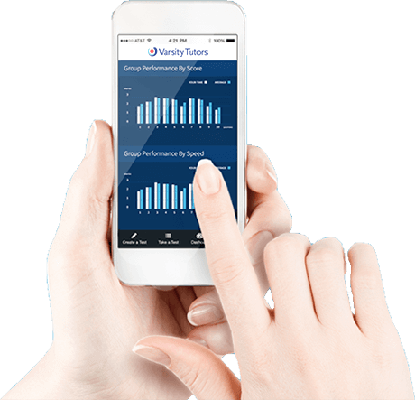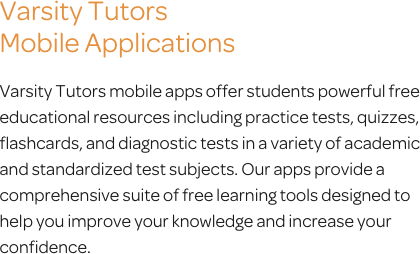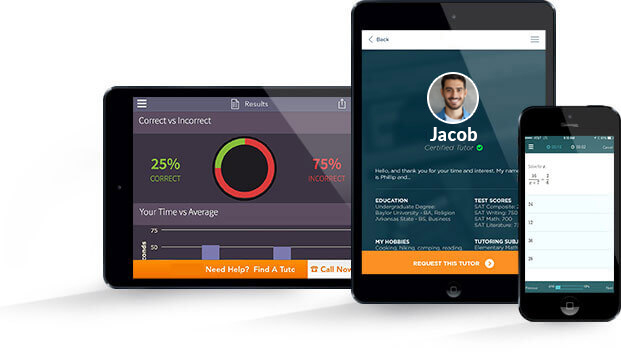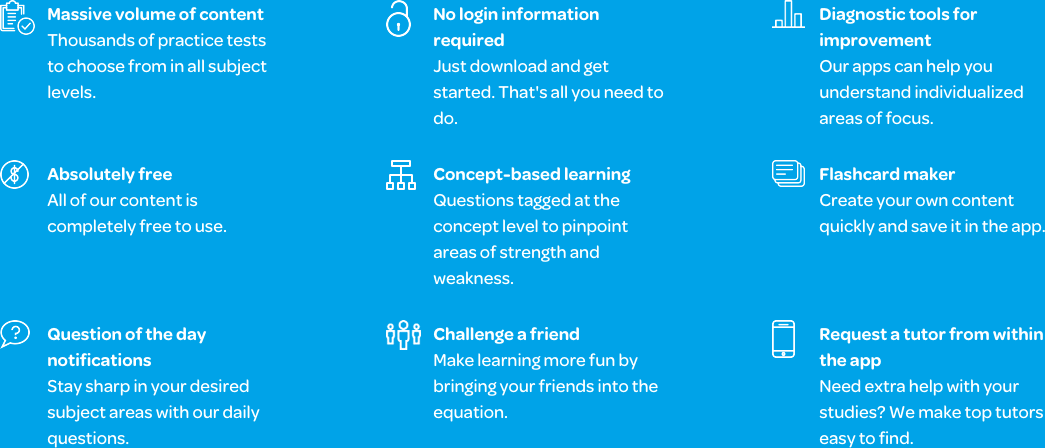# Varsity Tutors High School Math mobile app### The Varsity Tutors High School Math Mobile App

During high school, your child will be introduced to fundamental concepts in math, such as inequalities, coordinate geometry, and factoring. They may also dig deeper into higher-level math skills, like differentiation and integration. Many students struggle to fully understand math, and some need additional help to build a strong foundation of knowledge and logical reasoning. In order to progress to a successful future in post-secondary education and the professional world, students need to master these skills. Those who are seeking extra help may find the free Varsity Tutors High School Math app for Apple and Android devices useful. They can explore the challenges offered by calculus, basic algebra, trigonometry, and many other fields of math.

Through the app’s Learn by Concept resource, students are able to focus their studies on specific concepts. There are practice questions available that mimic what they may see on a real test to help them refresh their skills on specific concepts whenever they want. These are stress-free, and provide a full explanation of the correct answer to help your child understand more than just how to solve the problem.

Your child can prepare for a mid-term or final by taking multiple practice tests on a variety of math subjects, including pre-algebra, algebra, geometry, trigonometry, and calculus. Each practice test offers a great deal of information about your child’s performance. The full-length practice tests even highlight how your student scored in specific concept areas, and offers detailed explanations for the questions he or she may have missed. With these and the many other tools available on the app, students are able to customize their study sessions to ensure they are reviewing only the information they choose.

The app’s Question of the Day feature spontaneously tests students on various concepts involved in high school math. The question changes every 24 hours, and each question is pulled from one of the app’s many practice tests. Once the student answers the question, they’ll receive a review of their results compared to the others who answered the question. They can see how quickly they answered, how well they did in comparison to previous daily questions, and all of the other helpful metrics available on the results pages.

There is also an extensive library of flashcards available on the app, which allow students to fit in some study time on the bus or during passing periods. The Flashcard Maker enables students to design their own flashcards by uploading text, images of practice problems, or audio. With the flexibility of flashcards, it doesn’t matter how busy of a schedule your child has. They can work in studying during any amount of free time, while still sticking to the categories in which they need additional review.

To fully understand the abstract concepts of math, students need to put in additional time and effort outside the classroom. The Varsity Tutors High School Math app is a useful resource that can help guide your student alongside their ongoing classwork. Through the app, they have the opportunity to hone their critical thinking and logic skills. Available from the Google Play Store and iTunes, this free tool provides the help students need to grow confident in their skills before any upcoming exams.No matter who you are, you are going to cover many of the topics in high school math at some point during your educational experience. High school math spans several mathematical courses depending on your grade level and chosen diploma pathway. Most high school math curriculum takes you through algebra I, geometry, algebra II, and precalculus, while some will also introduce calculus. Math classes are important stepping stones that build your overall mathematical capabilities over the years, allowing you to enter college-level math courses that are more.

Depending on your grade, your high school math may vary. If you were able to complete algebra I during middle school, your first high school math class would be geometry. This is followed by algebra II, precalculus, and then calculus, with the option of taking an extra math class in statistics. Several of these courses can be taken at an AP level as well.

At the end of each high school math class, you are given a final exam that covers everything you discussed during the course. Since many math courses build upon existing knowledge from the previous one, there are often test questions that focus on your past experiences with math. You may be asked to answer a question that requires you to demonstrate your knowledge of quadratic equations; tackle radicals and exponents; solve for surface area, angles, distances, and lengths; identify real numbers; form operations using graphing and polynomial functions; and more. The amount of time you have to complete the test varies between schools, though many math-section exams have a full day to be completed.

The first level of high school math is algebra I. There are many things that you will learn during the class that will be valuable to future math courses. You will be introduced to important concepts such as identifying real numbers, working with linear and nonlinear equations, solving for one unknown variable, and using the systems of equations to solve problems. You will need to be able to choose the right equations to use to solve different problems, such as exponential, polynomial, factoring, and quadratic equations. In addition, you will be introduced to radical numbers, their functions, and how they can be simplified.

Depending on your school, liberal arts math may be a required part of the math curriculum, or it may be reserved for students that need additional work to progress. During liberal arts math, the class builds further on the knowledge you acquired in algebra I to provide a stronger basis for geometry. You will work with quadratic and linear equations; algebraic expressions; exponents, radicals, and polynomials; and linear inequalities and equalities. In addition, you will learn to solve problems that involve multiple dimensions, identify the principles of each triangle type, solve for volume and surface area, and work with coordinate geometry.

A geometry course is the next step in high school math. During your geometry class, you are introduced to basic graphing and forms of measurement. In addition, you will learn how to interact with compound loci through point sets, hyperbola, parabola, and ellipses. You will learn about different geometrical planes that are used to form area, arcs, radius, angles, and other important aspects of shapes. You will need to be able to solve the area for shapes such as ellipses, rectangles, sectors, parallelograms, trapeziums, circles, squares, and triangles. You will learn about the tools used to calculate areas, work with irregular polygons, identify the properties of a regular polygon, and construction for bisectors, line bisectors, and other segments.

Upon completing geometry, you can progress into algebra II. The skills and strategies you were introduced to in algebra I and geometry will be necessary to ensure success in this course. You will spend the term working with basic arithmetic operations using irrational numbers, nth roots, square roots, squares, and irrational numbers. You'll need to get a good understanding for denominators, Euler's numbers, the algebraic number, and transcendental numbers. You will learn about Coprimes, perfect numbers, Euclid's proof of endless prime numbers, Goldbach's conjecture, Mersenne primes, abundant numbers, amicable numbers, and deficient numbers. You must become familiar with Pythagorean triples, pi, the Liouville constant, and other transcendental numbers. You will work with complex numbers, such as negative square roots, imaginary numbers, and real numbers. Additional topics include conjugations, the fundamental theorem of algebra, and the units for the metric system. You will further need to demonstrate your ability to simplify radical expressions, perform basic arithmetic operations with radical expressions, identify the lowest terms in rational expressions, and solve rational inequalities. You will be expected to transform rational expressions into partial fractions, solve radical equations, work with fractional exponents, evaluate exponential expressions, and graph exponential numbers.

Precalculus typically follows algebra II, though some schools may allow you to opt for an alternative math program. During precalc, you receive the primary background information needed to understand the concepts, issues, problems, and strategies that are used in calculus. During the course, you begin working with complex number functions, matrices, vectors, trigonometry, and more. You will learn how to take a complex rational expression and rewrite it as a simplified one, identify combinatorics and probabilities, and develop an understanding for Cartesian coordinates, parametric equations, and polar coordinates. This introductory class serves as the stepping stone from high school math to college-level math.

Calculus can be a challenging course. However, if you are prepared with a solid mathematical foundation, you should be prepared to tackle these complex equations. The class may be taken as an AP course as well. You will dive into material that is typically reserved for college-level students, such as analyzing graphical evidence; comprehending one and two-sided limits for functions; an understanding of integrals, derivatives, and the fundamental theorem of calculus; antidifferentiation; and L'Hopital's rule. These are critical concepts to develop an understanding of in order to progress onto more advanced college-level courses.

High school math introduces you to a variety of complicated concepts that you will need to be able to draw on during higher level programs. The rate at which these classes are completed vary, as not every student goes straight into algebra I upon entrance into high school, or finishes up with calculus. Regardless, it is important to pay attention during your high school math classes and stay on top of your studies. There are many key pieces of information that have future implications for your education and eventual career.